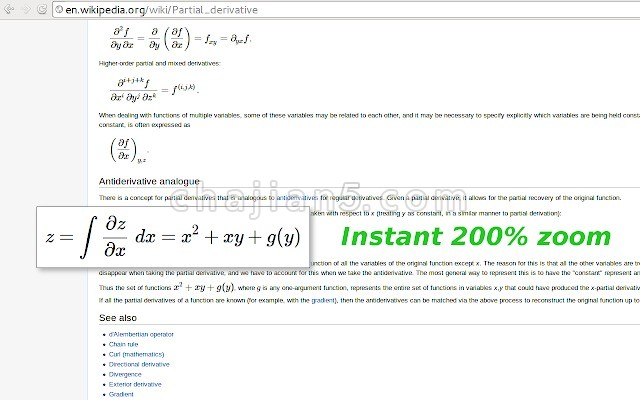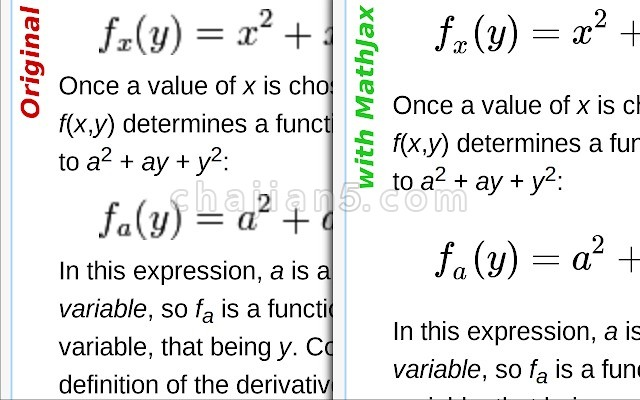• 为了带来更好的浏览体验，从6月8日起只保留插件的百度网盘、360云盘下载地址，感谢老铁们对本站一如既往的支持！

# 谷歌浏览器插件Wikipedia with MathJax 让维基百科支持数学公式显示Renders Wikipedia equations with MathJax
Extension replaces Wikipedia bitmap equations with HTML-CSS ones by re-rendering them with an open source MathJax (http://mathjax.org/) library. In effect, this allows to scale Wikipedia pages without loosing in equation quality.

Left-Click on equation to instantly zoom it to 200% (can be changed via MathJax menu).

Right-Click on equation to show MathJax’s context menu with additional options, e.g. “Scale All Math…” to instantly scale all equations on a page, “TeX commands” to see the source TeX equation etc.